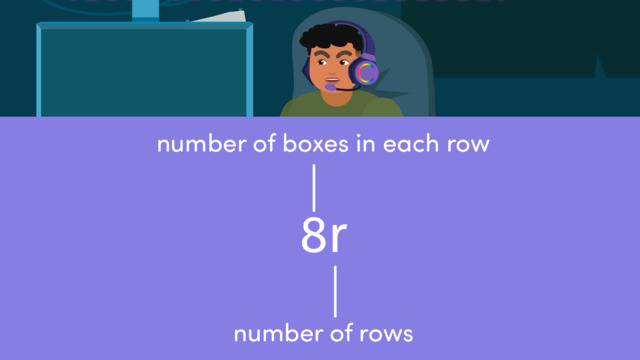# Using Variables to Represent Numbers in ExpressionsRating

Be the first to give a rating!
The authorsTeam Digital
Using Variables to Represent Numbers in Expressions
CCSS.MATH.CONTENT.6.EE.B.6

## Basics on the topicUsing Variables to Represent Numbers in Expressions

Let's learn about representing numbers with variables in expressions!

### TranscriptUsing Variables to Represent Numbers in Expressions

Using Variables to Represent Numbers in Expressions "Once I can buy this new headset I'm going to get so many 's. The surround sound and microphone clarity will be so much better, and.." "Yes, Uncle Mike! Wrapping up the cereal box count now!" Luis needs to calculate the number of cereal boxes in an efficient way. He could count them all by hand, but that might take a while. Using variables in expressions is an efficient approach to organizing information that has to be calculated. An expression is a combination of numbers, variables, and mathematical operations that can be evaluated to obtain a single value. Unlike an equation, it does not have an equal sign, it is simply a mathematical phrase that can be simplified or evaluated. A variable is a letter placeholder for an unknown number. The expression eight can help Luis calculate the number of cereal boxes because the display has EIGHT boxes in each row. So, EIGHT times represents the total number of cereal boxes. You may already know that even though there is no multiplication symbol, when a number and variable are next to one another it means to multiply. Luis moves over to the deli counter to make sandwiches. He puts cheese slices and pickle slices on a sandwich. He used five more pickle slices than cheese slices. If we use the variable to represent the cheese slice, which expression shows the number of pickle slices? (...) Using the expression plus five, we can determine the number of pickle slices on the sandwich based on the given information about the relationship between the number of cheese and pickle slices. Over in the bakery, Luis needs to share the total number of doughnuts he has left among THREE customers. If we use the variable to represent the doughnuts, which expression shows the number each customer will receive? (...) divided by three, because the total number of bagels is being DIVIDED among three customers. Before the end of his shift, Luis had to stock twelve milk containers, when all of sudden a some fell and exploded. What variable could we use to calculate the number of spilled milk containers? (...) While you can choose any letter you want for variables, it's useful to have one connected to your topic. If we use the variable to represent the spilled milk containers, which expression shows the number of milk containers left? (...) Twelve minus M represents how many milk containers are left. An expression is a combination of numbers, variables, and mathematical operations that can be evaluated to obtain a single value. A variable is a placeholder for an unknown number and a variable can be any letter, but it's helpful to choose a letter related to the expression. Variables in expressions are helpful in real life because they help us represent and understand unknown quantities or changing values in a way that makes solving problems and making calculations easier. It looks like Luis didn't waste any time crying over spilled milk.